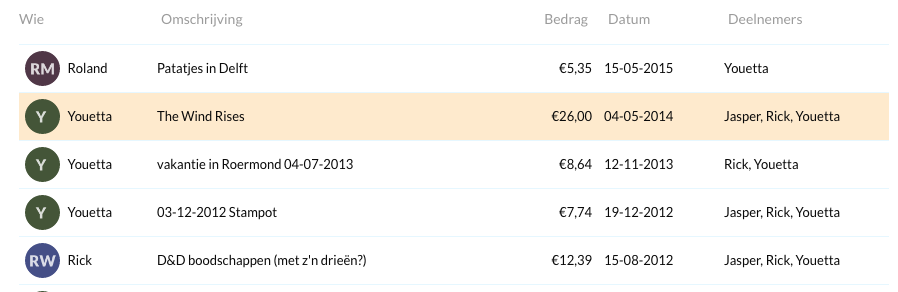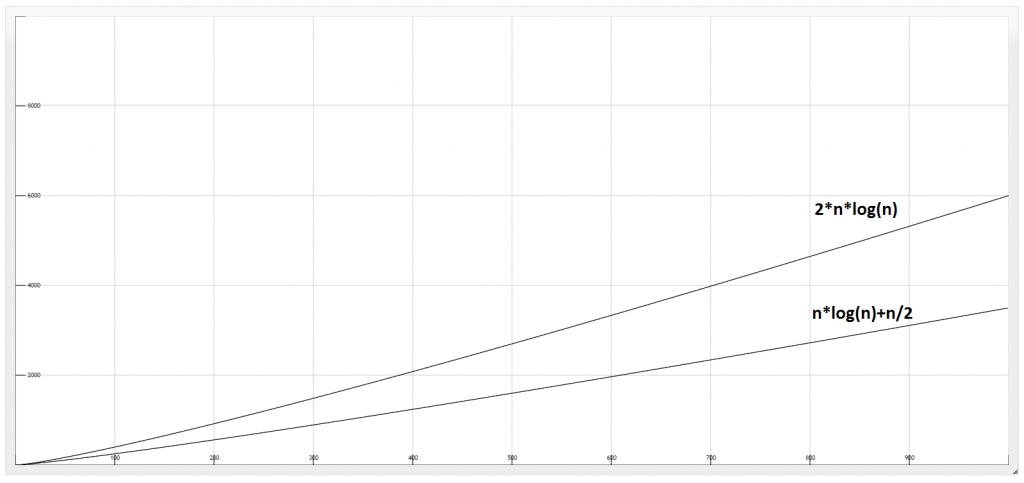# Calculating how to split your bills

Dutch people are known for splitting their bills. When frequently eating with the same group this could normally lead to an awkward situation, where everybody has to pay everybody a little bit. Most students use the site “https://wiebetaaltwat.nl/”. You create a group with the people you frequently eat with, and every time you do something with a set of these people you add your expense to the list.Once in a while you want your bills paid, to do this wiebetaaltwat tries to find a way in which there is the lowest amount of transactions. Last week I had a discussion with a friend about what would be the best way to calculate these transactions. This post will show my first attempt at finding an algorithm, why my algorithm was wrong, and how I improved it.

My first idea was:

• Input: a list L with the amount every person has to pay
• Returns: amount of transactions
• Define variable transaction_count
• while there is still an amount left:
• Sort L
• Let the person who has to pay the most (last element of L) pay to the person who has to get the most (first element of L)
• transaction_count +=1
• return transaction_count

Now every step either:

• Both amounts cancel each other
• The one who paid has no debt anymore
• The one who got money got everything he had to get

This guarantees that we take a maximum of N-1 transactions (which is pretty cool). Let’s analyse the complexity of this algorithm:

• The initial sorting takes n*log(n)
• One transaction calculation is O(1) (a step we have to take n times)
• Inserting the leftover value is log(n) (a step we have to take n times)

So this program has a complexity of n*log(n), it takes exactly n*log(n) + n/2 steps.

I programmed this out in Python to show that the approach works:

My friend wasn’t convinced by this script simply saying that it isn’t a good “real world example”. After a few hours I came up with an example that is NOT solved efficiently by my script:

Person Anna Bob Charlie Dennis Elisabeth
Initial money: 35 34 -2 -33 -34
E pays A 1 34 -2 -33 0
D pays B 1 1 -2 0 0
C pays A 0 1 -1 0 0
C pays B 0 0 0 0 0

How it should have been solved:

Person Anna Bob Charlie Dennis Elisabeth
Initial money: 35 34 -2 -33 -34
E pays C 35 0 -2 -33 0
D pays A 2 0 -2 0 0
C pays A 0 0 0 0 0
Nothing!! 0 0 0 0 0

As you can see by searching for the same value the amount of transactions can be reduced.
An observation we make is that this is done by removing values (a,b) where a==-b.
To do this we:

• Before each step, take a as the last element of L and b as the first element of L. Check if -a or -b is in L. If this is the case, merge these two.

Searching for two numbers in a list takes 2*log(n). With this update the total steps we take is n*log(n) + n/2 * 2 * log(n) which is 2*n*log(n).

I plotted the two graphs so you can see that the optimal solution takes double the time than my first solution:Is my second algorithm correct? Is there a way to calculate who has to pay what which leads to less transactions? I hope so! If you have a better algorithm, please leave a comment!